### Home > PC > Chapter 2 > Lesson 2.1.1 > Problem2-14

2-14.
1. Below are two of the special angles that are used in the unit circle. Identify the radian measure for the angles shown. Remember counter-clockwise angles are positive and clockwise angles are negative. Homework Help ✎

1.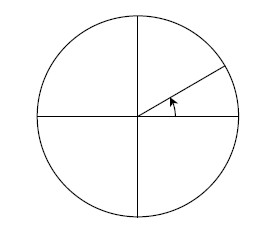2.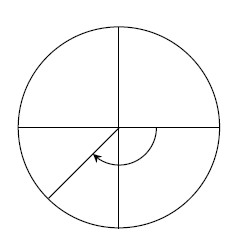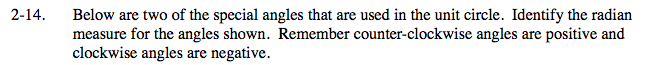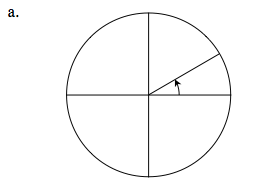$\frac{\pi}{6}$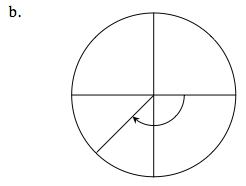$-\frac{3\pi}{4}$